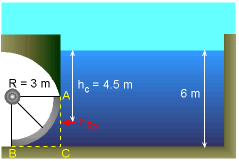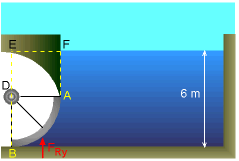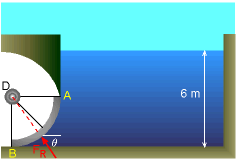Ch 2. Fluid Statics Multimedia Engineering Fluids PressureVariation PressureMeasurement HydrostaticForce(Plane) HydrostaticForce(Curved) Buoyancy
 Chapter 1. Basics 2. Fluid Statics 3. Kinematics 4. Laws (Integral) 5. Laws (Diff.) 6. Modeling/Similitude 7. Inviscid 8. Viscous 9. External Flow 10. Open-Channel Appendix Basic Math Units Basic Equations Water/Air Tables Sections Search eBooks Dynamics Fluids Math Mechanics Statics Thermodynamics Author(s): Chean Chin Ngo Kurt Gramoll ©Kurt GramollFLUID MECHANICS - CASE STUDY SOLUTIONX-Component of the Resultant Force In order to determine the magnitude of the resultant force exerted on the curved surface AB, first find its force components FRx and FRy. The horizontal projection of the curved surface AB is the plane area AC. The x-component of the resultant force is given by the normal force acting on this plane area. That is,      FRx = ρghc AAC            = (1000 kg/m3) (9.8 m/s2) (4.5 m) (3 m) (8 m)            = 1,058 kN Note that hc is the vertical distance to the centroid of plane area AC.Y-Component of the Resultant Force The y-component of the resultant force is the weight of the water directly above the curved surface (i.e., imaginary volume ABEF).      FRy = ρg VolABEF = ρg (VolADEF + VolABD)            = (1000 kg/m3) (9.8 m/s2) [(3 m) (3 m) (8 m)                  + (π 32/4) m2 (8 m) ]            = 1,260 kN Hence, the resultant force is given by      FR = (FRx2 + FRy2)0.5           = [(1,058 kN)2 + (1,260 kN)2]0.5           = 1,645 kNResultant Force and its Line of Action And the angle θ is given by      θ = tan -1 (FRy / FRx)         = tan -1 (1,260 kN / 1,058 kN)         = 50o Also, the resultant force FR has to pass through point D since all the pressure forces are perpendicular to the curved surface.

Practice Homework and Test problems now available in the 'Eng Fluids' mobile app
Includes over 250 problems with complete detailed solutions.
Available now at the Google Play Store and Apple App Store.